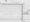# Mechanics of Solids Feb-2011

Unit -1

1.  a) The following data refer to a mild steel specimen tested in a laboratory.

i) Diameter of specimen = 25 mm

ii) Length of specimen = 300 mm

iii) Extension under a load of 15 kN = 0.045 mm.

iv) Load at yield point = 127.65 kN

v) Maximum load = 208.60 kN

vi) Length of specimen after failure = 375 mm.

vii) Neck diameter = 17.75 mm Determine the following:

i) Young’s Modulus

ii) Ultimate stress

iii )Yield pointPercentage reduction in area

v) Safe stress adopting a factor of safety of 2.

b) Find the extension of a bar uniformly tapering from diameter ‘d] ’ at one end to diameter ‘d2’ at other end subjected to an axial tensile load P at both ends. Length of bar is taken as ‘L’.

OR

a)  Show that relation between E, K, C. is given as Where : E = Young’s modulus K = Bulk modulus C = Modulus of rigidity.

b)  A steel bar is placed between two copper bars each having the same area and length as the steel bar at 15°C. At this stage they are rigidly connected together at both the ends. When the temperature is raised to 315°C, the length of the bar increases by 1.50 mm. Determine the original length and the final stresses in the bars. Take E = 2.1 * 105 N/mm2; E = 1 x 105 N/mm2. C 7 C(10) ac = 0.0000175 per °C as = 0.000012per °C.

Unit-II

2.a) Draw shear force and bending moment diagrams for the cantilever shown in fig.

b) A cast iron beam 2.75 m long has one support at the left end and the other support at 0.75 m from the right end. The beam is of T section consisting of a top flange 150 mmx20 mm and a web 20 mm wide and 80 mm deep. If the tensile stress and compressive stresses are not to exceed 40 N/mm2 and 70 N/mm2 resp. Find the safe concentrated load W that can be applied at the right end of the beam.

OR

a)  A beam 10 m long has supports at its ends A and B. It carries a point load of 5 kN at 3 m from A and a point load of 5 kN at 7 m from A and a uniformly distributed load of 1 kN per metre between the point loads. Draw load diagram, SFD and BMD for the beam.

b) Find the reactions at the fixed end of the cantilever loaded as shown in fig. Draw also the SFD and BMD.

Unit-III

3. (a) The intensity of resultant stress on a plane AB (as shown in fig ) at a point in the material under stress is 80 N/mm2 and is inclined at 30° to the normal to that plane. The normal component of stress on another plane BC. at right angles to the plane AB is 60 N/mm2. Determine the following :

i)The resultant stress on the plane BC.

ii)The principal stresses and their directions.

iii)The maximum shear stresses and their planes. 60 N/mm2b) Explain different theories of Elastic failures. Also explain their significance and applications.

OR

a)  At a point in a bracket the normal stresses on two mutually perpendicular planes are 120 N/mm2 (tensile) and 60 N/mm2 (tensile). The shear stress across these planes is 30 N/mm2. Find using Mohr’s circle, the principal stresses and maximum shear stress at the point.

b)  A tube of steel of 5 mm bore with a wall thickness of 0.5 mm is 1 metre long and is full of mercury. It is placed in horizontal position supported at its ends. If steel and mercury weigh 7.7×10′5 N/mm3 and 1.36><1 O’4 N/mm3 respectively. Find the maximum stress in the tube.

Unit-IV

4.a) What do you mean by pure torsion? What are the assumptions made in theory of pure torsion? Prove the relation.

b) A bar of length 4 metres when used as a simply supported beam and subjected to a uniformly distributed load of 30 kN/m over the whole span deflects

i) both ends pin jointed

ii) one end fixed and other hanged

ii) Both ends fixed.

OR

a)  Two shafts of the same material are subjected to the same torque. If the first shaft is of solid circular section and the second shaft is of hollow section whose internal diameter is 2/3 of the outside diameter. Compare the weights of two shafts.

b)  What are the assumptions made in Euler’s theory? Also explain the limitations of Euler’s formula.

Unit-V

5. a) Explain in detail Maxwell’s theorem of reciprocal deflection.

b)  A cast iron beam 40 mm wide and 80 mm deep is simply supported on a span of 1.2 m. The beam carries a point load of 15 kN at the centre. Find the deflection at the centre. Take E = 108000 N/mm2.

c)   A cantilever 3 metre long carries a uniformly distributed load over the entire length. If the slope at the free end is 1°. Find the deflection at the free end.(4)

OR

a) A uniform metal bar has a cross-sectional area of 700 mm2 and a length of 1.5 with an elastic limit of 160 N/mm2. What is the proof resilience? Find also the maximum value of an applied load which may be suddenly applied without exceeding the elastic limit. Calculate the value of gradually applied load which may produce the same extension as that produced by above supinely applied load. Take E = 2x 105 N/mm2.

b) Figure shows a simply supported beam of uniform section whose moment of inertia is 4.3 * 108mm4. For the loading shown, find the position and magnitude of maximum deflection. Take E = 200 kN. per mm2.